# Cylinder coordinates

cylindrical coordinates

Numbers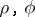andconnected with the Cartesian coordinatesandby the formulas: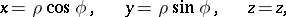where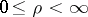,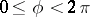,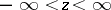. The coordinate surfaces (see Fig.) are: circular cylinders, half-planes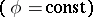and planes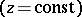.Figure: c027600a

The system of cylinder coordinates is orthogonal.

The Lamé coefficients are: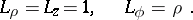The area element of a surface is: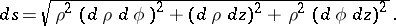The volume element is:The differentiation operations of vector analysis are given by: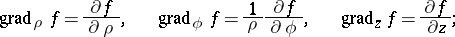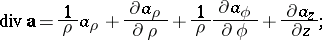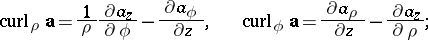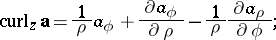Generalized cylinder coordinates are numbers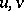and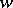connected with Cartesian coordinatesandby the formulas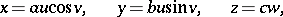where,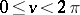,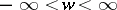,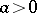,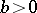,,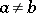. The coordinate surfaces are: elliptic cylinders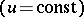, half-planesand planes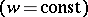.